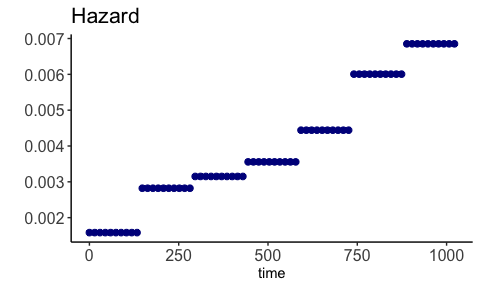# Introduction to package BayesSurvival

library(BayesSurvival)
library(survival)
library(ggplot2)

This package is suitable for unadjusted analyses of right-censored survival data. The samplers in this package allow for the computation of the posterior mean for the:

• hazard function;
• cumulative hazard function;
• survival function,

as well as credible bands for the:

• cumulative hazard function;
• survival function.

These credible bands provide reliable uncertainty quantification over the entire time interval (in contrast to guarantees for only a single time point), as justified in the paper Multiscale Bayesian Survival Analysis’ by Castillo and Van der Pas (2021+), available here: https://arxiv.org/abs/2005.02889. In the paper, one can also find the assumptions on the hazard under which the theory works.

The package offers the choice between two samplers:

• dependent Gamma prior;
• independent Gamma prior.

Both are instances of piecewise exponential priors, where the prior on the hazard function is taken to be piecewise constant. This vignette consists of three sections:

1. Details about the priors;
2. Example data analysis with the dependent Gamma prior;
3. Example data analysis with the independent Gamma prior.

Sections 2 and 3 provide a guided tour of the main functions of the package. Most users will only need the functions BayesSurv() and PlotBayesSurv(). Section 1 specifies which priors are implemented.

## 1. Details about the priors

### 1.1 Dependent Gamma prior

With Gamma($$\alpha$$, $$\beta$$), we refer to the Gamma distribution with shape parameter $$\alpha$$ and rate parameter $$\beta$$. Its density may be written as $$f_{\alpha, \beta}(x) = \frac{\beta^\alpha}{\Gamma(\alpha)}x^{\alpha-1}e^{-\beta x}$$.

The prior is piecewise constant and defined on the hazard $$\lambda$$. The time interval under study, denoted by $$[0, \tau]$$, is divided into $$K$$ intervals of equal size, denoted by $$I_k, k = 1, \ldots, K$$. The prior on $$\lambda$$ may be written as

$\lambda(t) = \sum_{k=1}^K \lambda_k \mathbf{1}\{t \in I_k\},$ where $$\lambda_1$$ is drawn from a Gamma($$\alpha_0, \beta_0$$) distribution and $$\lambda_k$$, for $$k = 2, \ldots, K$$, is drawn from a Gamma($$\alpha$$, $$\alpha/\lambda_{k-1}$$) distribution. The parameters $$\alpha_0, \beta_0, \alpha > 0$$ can be set by the user. The dependent structure of the prior is reflected by the prior mean and variance:

$E[\lambda_k \mid \lambda_{k-1}, \ldots, \lambda_1] = \lambda_{k-1};$ $Var(\lambda_k \mid \lambda_{k-1}, \ldots, \lambda_1) = \left(\frac{\lambda_{k-1}}{\alpha}\right)^2,$ for $$k = 2, \ldots, K$$.

For the number of intervals $$K$$, the default recommendation is to set $$K = \lceil (\tfrac{n}{\log{n}})^{1/2}\rceil$$, where $$n$$ is the sample size. If something is known about the smoothness of the underlying hazard, smaller values of $$K$$ may be selected. We refer the reader to the paper, Castillo and Van der Pas (2021+), for details about the selection of $$K$$ and for details about the MCMC algorithm implemented in this package.

### 1.2 Independent Gamma prior

The information for the independent Gamma prior is almost the same as for the dependent Gamma prior, with one key difference: each $$\lambda_k$$ is drawn independently from a Gamma($$\alpha$$, $$\beta$$) distribution.

## 2. Example data analysis with the dependent Gamma prior

We illustrate the main functions of the BayesSurvival package by performing a data analysis of the lung cancer data set from the survival package.

We first load the data and recode the status indicator, as the BayesSurv() function we will be using, will expect a 0 for censored individuals and a 1 for individuals whose survival time was observed.

cancer$status[cancer$status == 1] <- 0 #censored
cancer$status[cancer$status == 2] <- 1 #died

table(cancer$status) #> #> 0 1 #> 63 165 We now perform the unadjusted Bayesian analysis with the dependent Gamma prior. The main function of the package is BayesSurv() and that is where we will start. res <- BayesSurv(df = cancer, #our data frame time = "time", #name of column with survival/censoring times event = "status", #name of column with status indicator prior = "Dependent", #use dependent Gamma prior ) In the specification above, default values are used for the number of intervals $$K$$ and for the parameters $$\alpha_0, \beta_0$$ and $$\alpha$$. The default is to set $$K = \lceil (\tfrac{n}{\log{n}})^{1/2}\rceil$$, $$\alpha_0 = 1.5, \beta_0 = 1, \alpha = 1$$. Other values can be set by using the arguments K, alpha0.dep, beta0.dep and alpha.dep. The object res contains the posterior means for the hazard, cumulative hazard and the survival function. It also contains the radii for the $$(1-\alpha_{cb})$$%-credible bands for the cumulative hazard and for the survival function, with the level $$\alpha_{cb}$$ set to 0.05 by default. The argument alpha may be used for other values. The results can be visualized by using the PlotBayesSurv() function. By default, the posterior mean will be plotted, and (if applicable) the credible band as well. PlotBayesSurv(bayes.surv.object = res, object = "survival")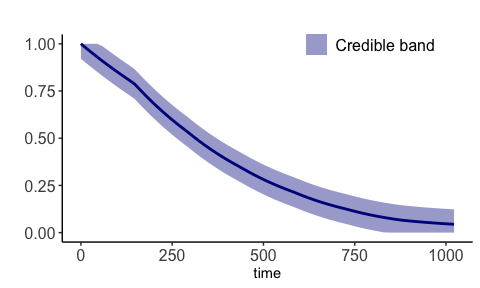There are some options to customize the graph. For example, one can add a title and change the color. PlotBayesSurv(bayes.surv.object = res, object = "survival", color = "orange", plot.title = "Survival function")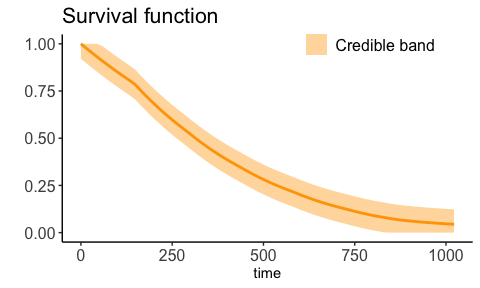The plots are made in ggplot2 and can be processed further using ggplot2 plotting options. For example, the Kaplan-Meier estimate of the survival function can be added. gg <- PlotBayesSurv(bayes.surv.object = res, object = "survival") km <- survfit( Surv(time, status) ~ 1, data = cancer ) #Kaplan-Meier df.km <- data.frame(t = km$time, km = km\$surv)

gg <- gg + geom_line(data = df.km, aes(x = t, y = km), colour = "black", size = 1, lty = 6)
gg <- gg + labs(title = "With Kaplan-Meier + CI's")
gg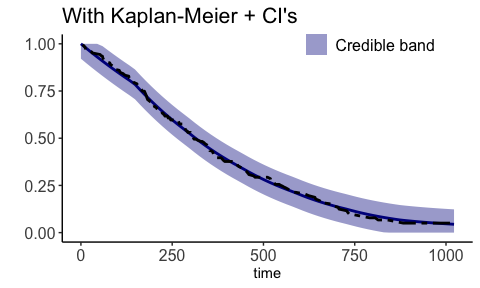A plot for the cumulative hazard is obtained similarly.

PlotBayesSurv(bayes.surv.object = res,
object = "cumhaz",
plot.title = "Cumulative hazard")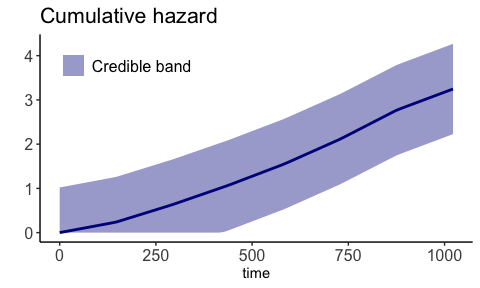And finally, the posterior mean of the hazard function may be visualized as well. No credible band is provided in this case.

PlotBayesSurv(bayes.surv.object = res,
object = "hazard",
plot.title = "Hazard")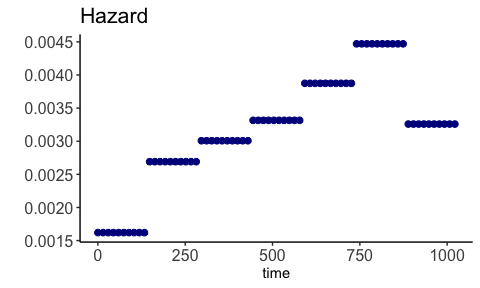## 3. Example data analysis with the independent Gamma prior

The analysis is very similar for the independent Gamma prior, compared to the dependent Gamma prior. The main difference is in the prior argument in BayesSurv(), where the following code could be used.

res <- BayesSurv(df = cancer, #our data frame
time = "time", #name of column with survival/censoring times
event = "status", #name of column with status indicator
prior = "Independent", #use independent Gamma prior
)

In the specification above, default values are used for the number of intervals $$K$$ and for the parameters $$\alpha$$, and $$\beta$$. The default is to set $$K = \lceil (\tfrac{n}{\log{n}})^{1/2}\rceil$$, $$\alpha = 1.5, \beta = 1$$. Other values can be set by using the arguments K, alpha.indep, and beta.indep.

The results for the independent Gamma prior can be processed in the same way as for the dependent Gamma prior. For example, the code below would lead to plots of the posterior means of the survival, cumulative hazard and hazard respectively. These plots can be customized in the same way as described for the dependent Gamma prior.

PlotBayesSurv(bayes.surv.object = res,
object = "survival",
plot.title = "Survival")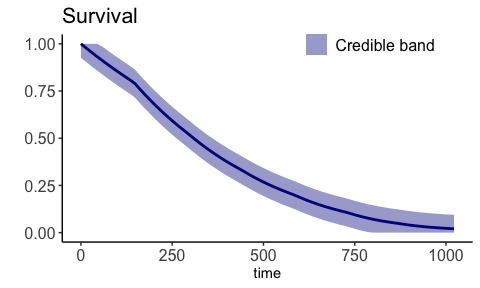PlotBayesSurv(bayes.surv.object = res,
object = "cumhaz",
plot.title = "Cumulative hazard")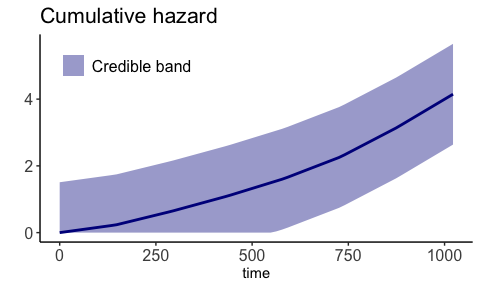PlotBayesSurv(bayes.surv.object = res,
object = "hazard",
plot.title = "Hazard")`